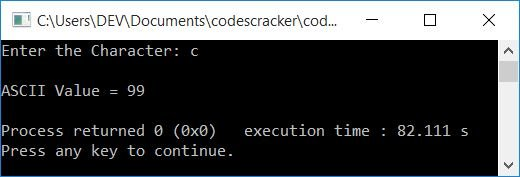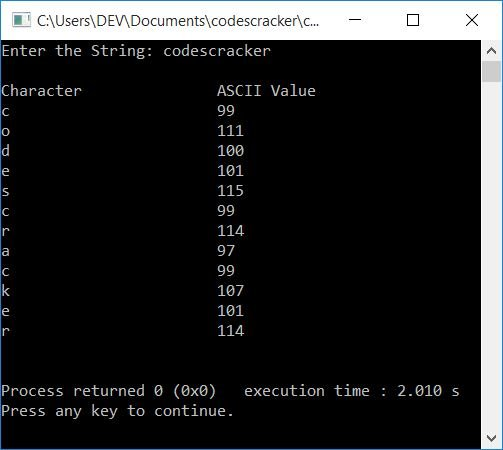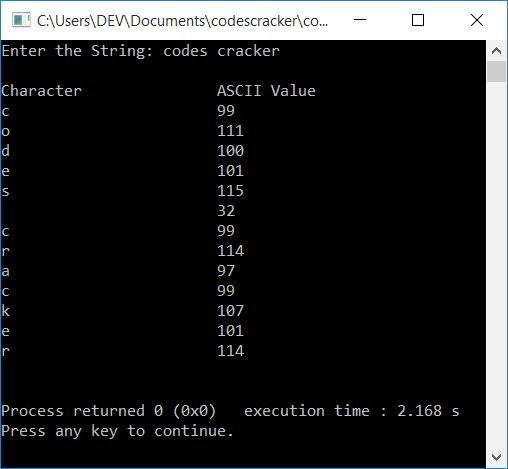# C++ program to print the ASCII value of characters

This article will teach you how to print the ASCII value of a character in C++ and provide you with code to do so. Here is the list of programs for printing ASCII values available in this article:

### What is ASCII?

ASCII stands for "American Standard Code for Information Interchange." It is a character encoding standard for electronic communication.

## Print a character's ASCII value in C++

This program receives a character as input from the user at run-time and prints its ASCII value.

```#include<iostream>
using namespace std;
int main()
{
char ch;
int i;
cout<<"Enter the Character: ";
cin>>ch;
i = ch;
cout<<"\nASCII Value = "<<i;
cout<<endl;
return 0;
}```

This program was built and runs under the Code::Blocks IDE. Here is its sample run:Now supply the input character, say "c," to find and print its ASCII value as shown in the snapshot given below:The following statement:

`i = ch;`

initialized the ASCII value (an integer value) of ch to i. Because the user entered c as a character input, c (the small letter) gets stored in ch, and using the above statement, the ASCII value of ch (99) gets stored in (or initialized in) i, because i is an int (integer) type variable. Now just print the value of i as the ASCII value of c on the output.

## In C++, print the ASCII values of all characters

To print the ASCII values of all characters in C++ programming, use a for loop to execute a block of code  255 times. That is, the loop variable i starts with 0 and ends with 255.

Every time the value of i gets initialized to ch, which is a char (character) type variable, the corresponding character to the value of i gets initialized to ch. Here, the value of i is considered an ASCII value. Therefore, if i's value is 65, then the character whose ASCII value is 65 gets initialized to ch, because 65 is the ASCII value of A. So at that time, ch = A.

Print ch as a character and the value of i as its ASCII value, each time while entering into the loop's body, as shown in the program given below:

```#include<iostream>
using namespace std;
int main()
{
char ch;
int i;
cout<<"Character\t\tASCII Value\n";
for(i=0; i<255; i++)
{
ch = i;
cout<<ch<<"\t\t\t"<<i<<endl;
}
cout<<endl;
return 0;
}```

Here is its sample output. The snapshot given below shows a small part of the sample output produced by this program:## Print the ASCII code for each character in a string

This is the last program on printing ASCII values. This program receives a string input from the user and prints the ASCII value of each and every character of the given string.

```#include<iostream>
#include<stdio.h>
using namespace std;
int main()
{
char ch, str;
int i=0, val;
cout<<"Enter the String: ";
gets(str);
cout<<"\nCharacter\t\tASCII Value\n";
while(str[i])
{
ch = str[i];
val = ch;
cout<<ch<<"\t\t\t"<<val<<endl;
i++;
}
cout<<endl;
return 0;
}```

Here is its sample run with user input, codescracker:Here is another sample run with user input, codes cracker:#### The same program in different languages

C++ Quiz

« Previous Program Next Program »